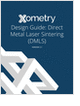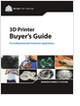MaterialsDesign CenterProcessesUnit ConversionFormulasMathematicsCalculatorsDiscussion ForumTrade PublicationsDirectory Service

Learn the best principles to negotiate the salary you deserve!

Metal 3D Printing Design Guide

Direct Metal Laser Sintering (DMLS) 3D printing for parts with reduced cost and little waste.

Selecting the Right 3D Printer

Discover how to choose the right 3D printer for your needs and the key performance attributes to consider.

Salary Expectation

8 things to know about the interview question "What's your salary expectation"?

more free publicationsGlossary » Units » Pressure » Pound Force Per Square InchPound Force Per Square Inch (psi, PSI, lbf/in2) is a unit in the category of Pressure. It is also known as pound force/square inch, pound per square inch. This unit is commonly used in the UK, US, FPS unit systems. Pound Force Per Square Inch (psi, PSI, lbf/in2) has a dimension of ML-1T-2 where M is mass, L is length, and T is time. It can be converted to the corresponding standard SI unit Pa by multiplying its value by a factor of 6894.757292.
Note that the seven base dimensions are M (Mass), L (Length), T (Time), Q (Temperature), N (Aamount of Substance), I (Electric Current), and J (Luminous Intensity).

Other units in the category of Pressure include Atmosphere (metric) (at), Atmosphere (standard) (atm), Bar (bar), Barad (barad), Barye, CentiHg (0°C), Centimeter of Mercury (0°C) (cmHg (0 °C)), Centimeter of Water (4°C) (cmH2O), Dyne Per Square Centimeter (dyn/cm2), Foot of Water (4°C) (ft H2O), Gigapascal (GPa), Hectopascal (hPa), Inch of Mercury (0°C) (inHg (0 °C)), Inch of Mercury (15.56°C) (inHg (15.56 °C)), Inch of Water (15.56°C) (inH2O (15.56 °C)), Inch of Water (4°C) (inH2O (4 °C)), Kilogram Force Per Square Centimeter (kgf/cm2), Kilogram Force Per Square Decimeter (kgf/dm2), Kilogram Force Per Square Meter (kgf/m2), Kilogram Force Per Square Millimeter (kgf/mm2), Kilopascal (kPa), Kilopound Force Per Square Inch (kip/in2, ksi, KSI), Megapascal (MPa), Meter of Water (15.56°C) (mH2O, mCE (15.56 °C)), Meter of Water (4°C) (mH2O, mCE (4 °C)), Microbar (barye, Barrie) (µbar), Micron of Mercury (millitorr) (µHg (0 °C)), Millibar (mbar), Millimeter of Mercury (0°C) (mmHg, torr, Torr (0 °C)), Millimeter of Water (15.56°C) (mmH2O, mmCE (15.56 °C)), Millimeter of Water (4°C) (mmH2O, mmCE (4 °C)), Millitorr (mtorr), Newton Per Square Meter (N/m2), Ounce Force (av.) Per Square Inch (ozf/in2, osi), Pascal (Pa, N/m2), Pound Force Per Square Foot (lbf/ft2), Poundal Per Square Foot (pdl/ft2), Poundal Per Square Inch (pdl/in2), Standard Atmosphere (atm), Ton Force (long) Per Square Foot (tonf/ft2 (UK)), Ton Force (long) Per Square Inch (tonf/in2 (UK)), Ton Force (metric) Per Square Centimeter (tonf/cm2 (metric)), Ton Force (metric) Per Square Meter (tonf/m2 (metric)), Ton Force (short) Per Square Foot (tonf/ft2 (US)), Ton Force (short) Per Square Inch (tonf/in2 (US)), and Torr (torr).N/ARelated PagesGlossaryLearn the best principles to negotiate the salary you deserve!Metal 3D Printing Design Guide

Direct Metal Laser Sintering (DMLS) 3D printing for parts with reduced cost and little waste.Selecting the Right 3D Printer

Discover how to choose the right 3D printer for your needs and the key performance attributes to consider.Salary Expectation

8 things to know about the interview question "What's your salary expectation"?